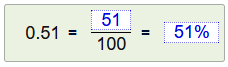Percentage   »A decimal number was created at the start of the page. Your task now is to write the decimal number as a fraction with the denominator 100. and then as a percentage. Example solution:Click on  new  to create a new problem.
Can you top 395 points?

#### Percentage calculationDecimal number and percent

Write as a fraction then in %
 =

realmath.de

... more than just practicing